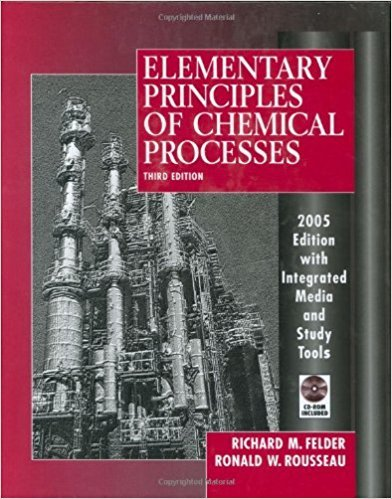×
Get Full Access to Elementary Principles Of Chemical Processes - 3 Edition - Chapter 14 - Problem 14.15
Get Full Access to Elementary Principles Of Chemical Processes - 3 Edition - Chapter 14 - Problem 14.15

×

# Assuming that option 2 in 14.14 is used, construct a graphISBN: 9780471687573 143

## Solution for problem 14.15 Chapter 14

Elementary Principles of Chemical Processes | 3rd Edition

• Textbook Solutions
• 2901 Step-by-step solutions solved by professors and subject experts
• Get 24/7 help from StudySoup virtual teaching assistantsElementary Principles of Chemical Processes | 3rd Edition

4 5 1 293 Reviews
26
3
Problem 14.15

Assuming that option 2 in 14.14 is used, construct a graph of the velocity of the stack gas (1 atm, 80C) versus the diameter of the stack. Cover a range of velocities from 5 to 15 m/s.

Step-by-Step Solution:
Step 1 of 3

Step 2 of 3

Step 3 of 3

##### ISBN: 9780471687573

The answer to “Assuming that option 2 in 14.14 is used, construct a graph of the velocity of the stack gas (1 atm, 80C) versus the diameter of the stack. Cover a range of velocities from 5 to 15 m/s.” is broken down into a number of easy to follow steps, and 37 words. The full step-by-step solution to problem: 14.15 from chapter: 14 was answered by , our top Chemistry solution expert on 11/15/17, 02:42PM. Since the solution to 14.15 from 14 chapter was answered, more than 353 students have viewed the full step-by-step answer. Elementary Principles of Chemical Processes was written by and is associated to the ISBN: 9780471687573. This full solution covers the following key subjects: Stack, option, construct, cover, diameter. This expansive textbook survival guide covers 13 chapters, and 710 solutions. This textbook survival guide was created for the textbook: Elementary Principles of Chemical Processes, edition: 3.

Unlock Textbook Solution